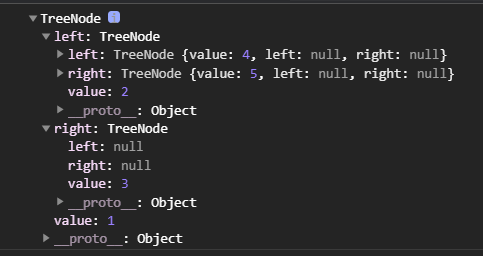# 以中序遍历为例

1、定义树节点

``````class TreeNode{

constructor(value){

this.value = value;
this.left = null;
this.right = null;
}
}
``````

2、构建树

``````// 构建树
let root = new TreeNode(1);
root.left = new TreeNode(2);
root.right = new TreeNode(3);
root.left.left = new TreeNode(4);
root.left.right = new TreeNode(5);
console.log(root);//如下图
``````## 递归算法

``````// 递归中序遍历二叉树
function midOrder(root) {

if(!root || !(root instanceof TreeNode)){

return;
}
//instanceof 运算符用于检测构造函数的 prototype 属性是否出现在某个实例对象的原型链上。
// 递归访问左子树
midOrder(root.left);
console.log(root.value);
// 递归访问右子树
midOrder(root.right);
}
midOrder(root);
``````

## 非递归算法

``````// 非递归中序遍历二叉树
function midOrderN(root) {

let p = root; // p为当前遍历的节点， 初始为根
let arr = []; // arr作为栈
while(p || arr.length !== 0){

if(p){

// 遍历左子树
arr.push(p);
// 每遇到非空二叉树先向左走
p = p.left;
}else{

// p为空，出栈
let node = arr.pop();
// 访问该节点
console.log(node.value);
// 向右走一次
p = node.right;
}
}
}
midOrderN(root)
``````

# 前序、中序、后序

## 递归写法

``````function TreeNode(x){

this.val=val;
this.left=null;
this.right=null;
}
// 递归方法
function threeOrders(root){

let preArray=[],middleArray=[],lastArray=[];
//先序遍历：根、左、右
function preOrder(root){

if(root){

preArray.push(root.val);
preOrder(root.left);
preOrder(root.right);
}
}
//中序遍历 ： 左 根 右
function inOrder(root){

if(root){

inOrder(root.left)
middleArray.push(root.val);
inOrder(root.right);
}
}
//后序遍历：左右根
function lastOrder(root){

if(root){

lastOrder(root.left);
lastOrder(root.right);
lastArray.push(root.val);
}
}
preOrder(root);
inOrder(root);
lastOrder(root);
return [preArray,middleArray,lastArray]
}

``````

## 非递归写法

``````function threeOrders(root){

//非递归算法实现先序遍历二叉树，根左右，所以向数组中push一个元素
function preOrder(root){

let res=[],
stack=[root];
while(stack.length>0){

let node=stack.pop();
res.push(node.val);
if(node.right){

stack.push(node.right);
}
if(node.left){

stack.push(node.right);
}

}
return res;
}
//非递归算法 实现中序遍历二叉树   首先遍历找到最深层的左子树，
function inOrder(root){

let res=[],
stack=[];
while(root||stack.length>0){

while(root){

stack.push(root);
root=root.left;
}
root=stack.pop();
res.push(root.val);
root=root.right;
}
return res;
}
// 非递归算法实现后序遍历二叉树， 和先序遍历二叉树类似，唯一区别是向数组中unshift元素，先push左再push右
function lastOrder(root){

let res=[],
stack=[root];
while(stack.length>0){

let node=stack.pop();
res.unshift(node.val);
if(node.left){

stack.push(node.left);
}
if(node.right){

stack.push(node.right);
}
}
return res;
}
}

``````

### LabVIEW LINX Toolkit控制Arduino设备（拓展篇—1）_labview spi接口_不脱发的程序猿的博客-程序员秘密

LabVIEW LINX Toolkit可支持驱动Raspberry Pi、BeagleBoard和Arduino开发板，包含数字、模拟、SPI、I2C、UART、PWM等驱动接口，非常适合创客开发实践。

### php7随机数,random_int()_倦与暮的博客-程序员秘密

random_int()(PHP 7)Generates cryptographically secure pseudo-random integers说明random_int(int\$min,int\$max):intGenerates cryptographic random integers that are suitable for use where unbiased results ar...

### RxJava开发精要7 - Schedulers-解决Android主线程问题_西门大官人很喜欢的博客-程序员秘密

RxJava开发精要7 - Schedulers-解决Android主线程问题

### (原創) Write once, compile everywhere? (C/C++) (SystemC) (VC++) (IC Design)_weixin_34344403的博客-程序员秘密

Abstract這兩天寫SystemC的第一個作業，其實花最多時間是在Compiler身上。由於SystemC本身並不是一個程式語言，而是架構在C++上，利用C++的Generics特性擴充其Library，使C++搖身一變成為HDL，且SystemC也沒有自己的IDE和Compiler，理論上只要是C++的Compiler就可以compile所有SystemC的code。Introducti...

### xp系统安装后只有一个盘了别的分区的文件怎样找到_weixin_34162401的博客-程序员秘密

GHOST系统分区丢失是因为在重装系统时，选择了错误的选项导致把整个硬盘当成C盘来装，装完之后自然就只剩下一个盘。想要恢复丢失盘的文件，需要注意，别往现在的这个C盘存入新的文件（因为现在存入的文件可能会覆盖原先DEF盘的文件）。可以把硬盘拆下来挂到别的电脑当副盘或者接个移动硬盘，把数据恢复到移动硬盘里。具体的恢复方法看下文了解。工具/软件：流星数据恢复软件步骤1：先下载并解压程序运行后，直接双...

### 稳步前行or大干快上——5G非独立组网与独立组网_tiege8066的博客-程序员秘密

*******更多精彩5G内容请打开链接https://edu.csdn.net/course/detail/311825G标准的发布，相当于3GPP画了一只色香味俱全、令人垂涎欲滴的大蛋糕。那么，谁来做这只蛋糕呢？是各国的移动通信运营商。但是，这款蛋糕好是好，成本可是太高了。因为5G网络大都工作在很高的频段，每个基站的覆盖半径很小，若要形成大范围的覆盖需要建设大量的基站。万一投入巨额资金做成了这只蛋糕，很少有人买来吃怎么办？运营商的钱岂不是打水漂了？3GPP里都是些什么人哪，都是业界的精英翘楚啊，人家

### python抢票代码_Python实现12306火车票抢票系统_weixin_39849548的博客-程序员秘密

Python实现12306火车票抢票系统效果图如下所示：具体代码如下所示：import urllib.request as requestimport http.cookiejar as cookiejarimport reimport osimport smtplibfrom email.mime.text import MIMETextimport timeuser = '' #登陆邮箱pwd...